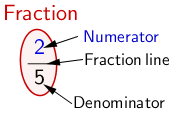Two fractions A and B of a meter are given. Task: Move the sliders -- Denominator    -- Numerator -- Denominator    -- Numerator and create the corresponding drawings.

Click on  new  to create a new problem.

Can you top 299 points?

#### Draw fractions of a meter• Designationsspeak: "Two fifths" ‣ The denominator tells how many equal parts the whole is divided into. ‣ The numerator tells how many of these parts are taken. ‣ Numerator and denominator are separated by the fraction line.